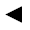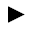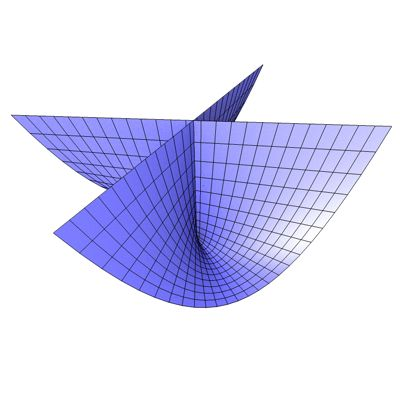﻿﻿﻿ math handbook calculator - Fractional Calculus Computer Algebra System software+++=﻿[back]

## 3.18. Whitney Umbrella

[next]The Whitney Umbrella [ 6,11,18 ] is represented by the following equations.

 x = uv 3-68 y = u 3-69 z = v2 3-70

To represent the area, the two parameters u and v can assume the following values ​​(domain of definition), for example.

 u is an element from the set of numbers [-1.5, 1.5] v is an element from the set of numbers [-1.5, 1.5]

The Whitney Umbrella is an open figure, so the definition range can be changed in the plugin.Fig. 22